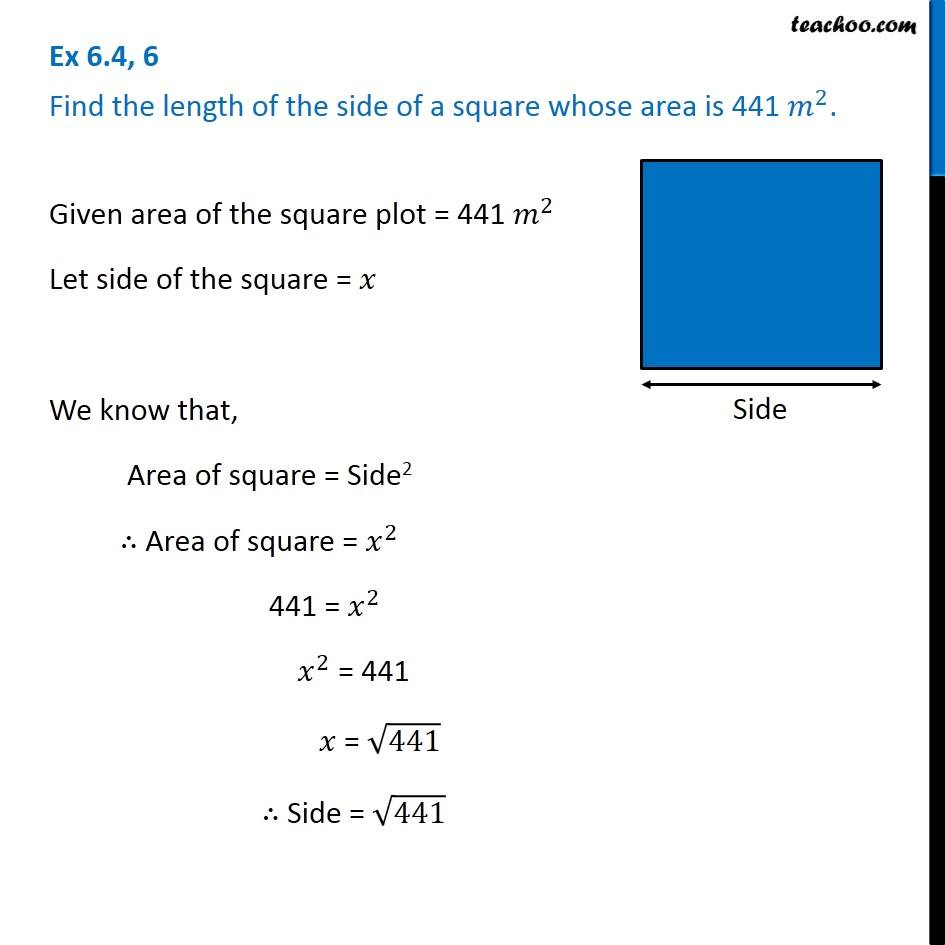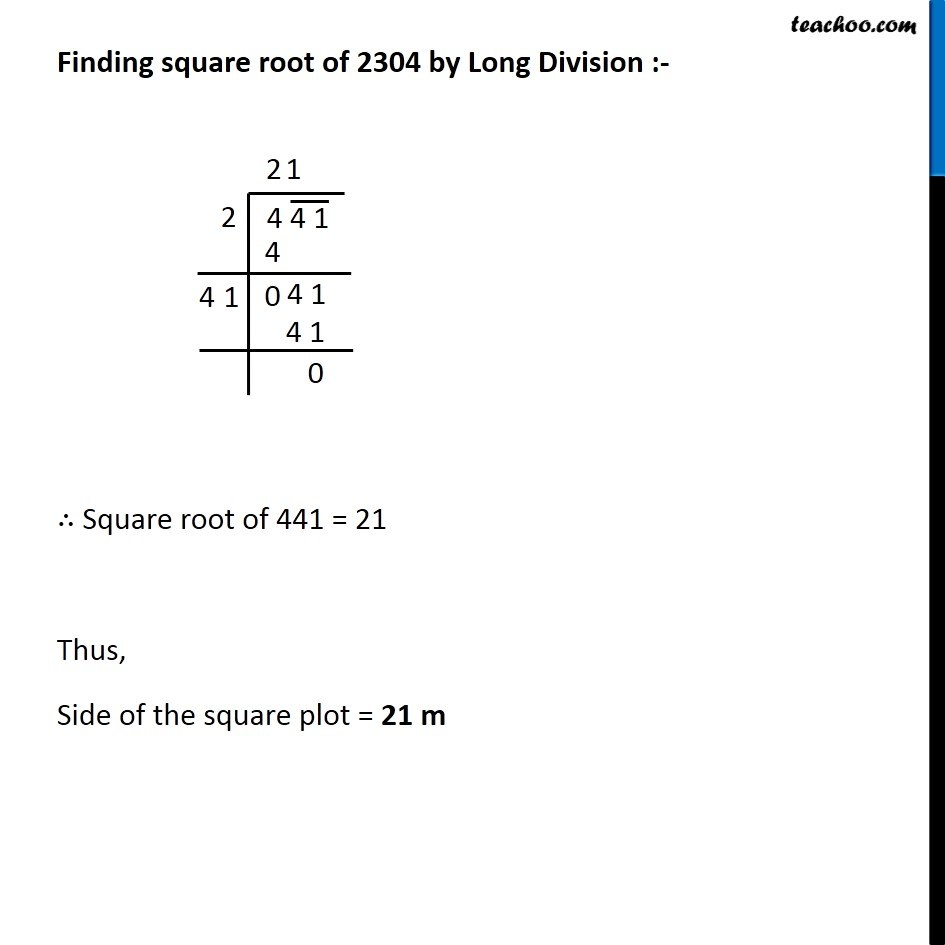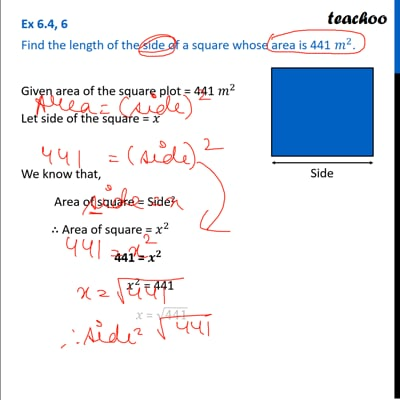Statement Questions

Chapter 6 Class 8 Squares and Square Roots
Concept wiseThis video is only available for Teachoo black users

Get live Maths 1-on-1 Classs - Class 6 to 12

### Transcript

Ex 6.4, 6 Find the length of the side of a square whose area is 441 𝑚^2.Given area of the square plot = 441 𝑚^2 Let side of the square = 𝑥 We know that, Area of square = Side2 ∴ Area of square = 𝑥^2 441 = 𝑥^2 𝑥^2 = 441 𝑥 = √441 ∴ Side = √441 Finding square root of 2304 by Long Division :- ∴ Square root of 441 = 21 Thus, Side of the square plot = 21 m